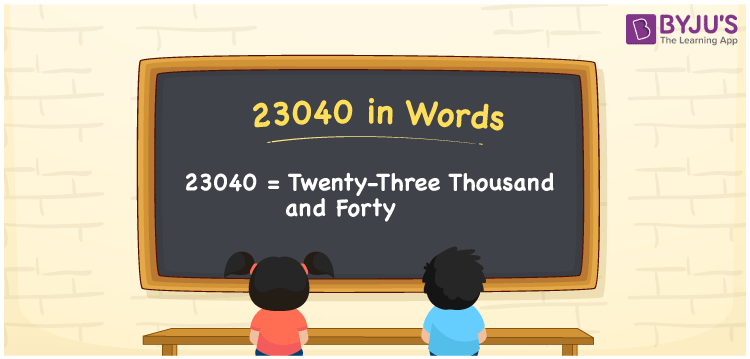# 23040 in words

23040 in words is written as Twenty Three Thousand and Forty. In 23040, 2 has a place value of ten thousand, 3 is in the place value of thousand and 4 is in the place value of ten. The article on Place Value gives more information. The number 23040 is used in expressions that relate to money, distance, social media views, and many more. For example, “The postal code for Cumberland, USA, is Twenty Three Thousand and Forty.”

 23040 in words Twenty Three Thousand and Forty Twenty Three Thousand and Forty in Numbers 23040

## 23040 in English Words## How to Write 23040 in Words?

We can convert 23040 to words using a place value chart. The number 23040 has 5 digits, so let’s make a chart that shows the place value up to 5 digits.

 Ten thousand Thousands Hundreds Tens Ones 2 3 0 4 0

Thus, we can write the expanded form as:

2 × Ten thousand + 3 × Thousand + 0 × Hundred + 4 × Ten + 0 × One

= 2 × 10000 + 3 × 1000 + 0 × 100 + 4 × 10 + 0 × 1

= 23040.

= Twenty Three Thousand and Forty.

23040 is the natural number that is succeeded by 23039 and preceded by 23041.

23040 in words – Twenty Three Thousand and Forty.

Is 23040 an odd number? – No.

Is 23040 an even number? – Yes.

Is 23040 a perfect square number? – No.

Is 23040 a perfect cube number? – No.

Is 23040 a prime number? – No.

Is 23040 a composite number? – Yes.

## Solved Example

1. Write the number 23040 in expanded form

Solution: 2 x 10000 + 3 x 1000 + 0 x 100 + 4 x 10 + 0 x 1

Or Just 2 x 10000 + 3 x 1000 + 4 x 10

We can write 23040 = 20000 + 3000 + 000 + 40 + 0

= 2 x 10000 + 3 x 1000 + 0 x 100 + 4 x 10 + 0 x 1.

## Frequently Asked Questions on 23040 in words

Q1

### How to write the number 23040 in words?

23040 in words is written as Twenty Three Thousand and Forty.
Q2

### State whether True or False. 23040 is divisible by 3?

True. 23040 is divisible by 3.
Q3

### Is 23040 divisible by 10?

Yes. 23040 is divisible by 10.